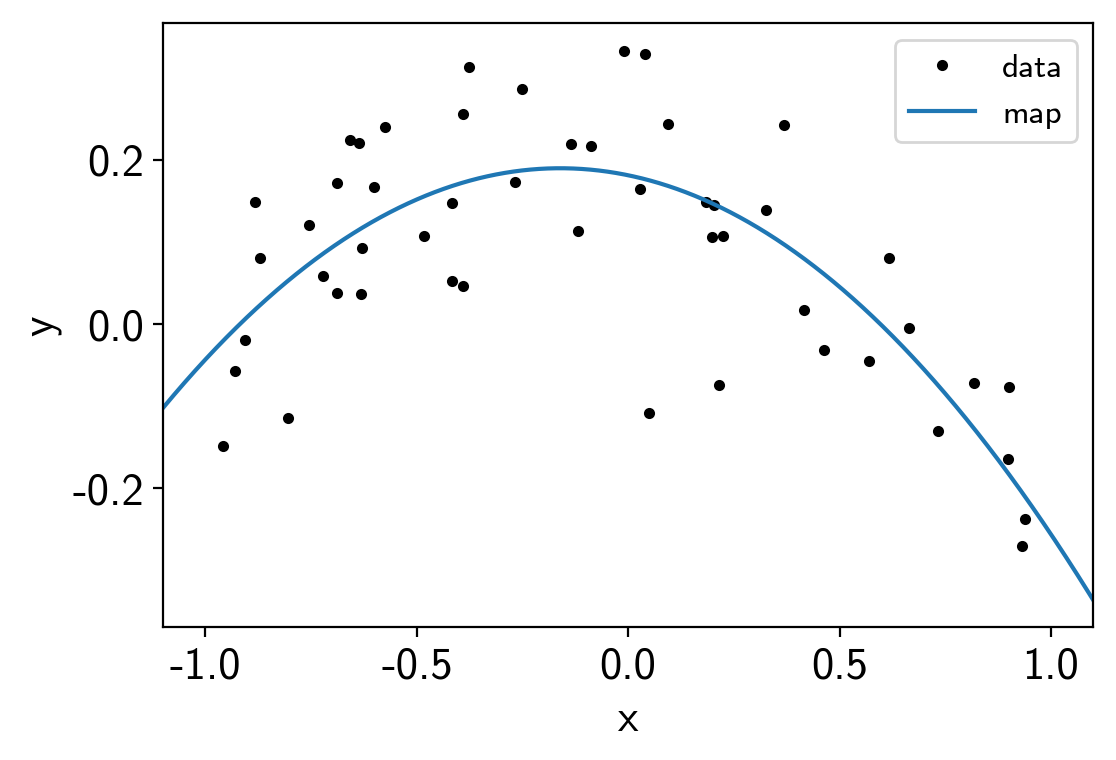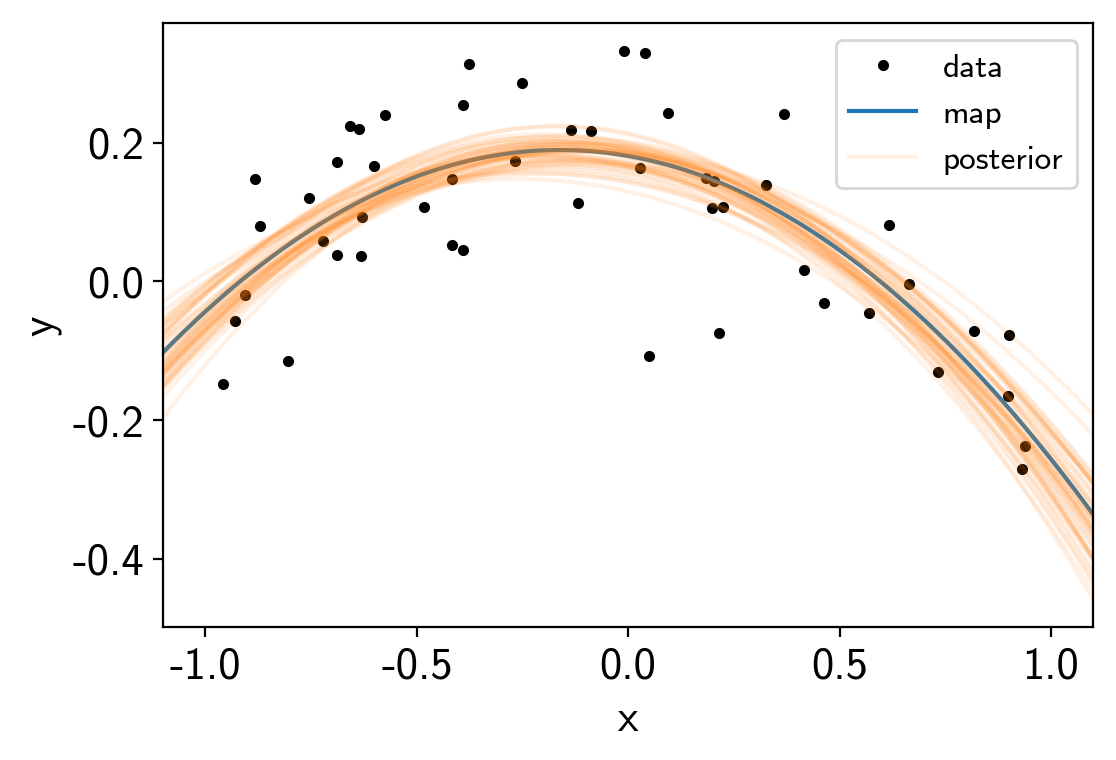Note

This tutorial was generated from an IPython notebook that can be downloaded here.

# PyMC3 extras¶

exoplanet comes bundled with a few utilities that can make it easier to use and debug PyMC3 models for fitting exoplanet data. This tutorial briefly describes these features and their use.

## Custom tuning schedule¶

The main extra is the exoplanet.PyMC3Sampler class that wraps the PyMC3 sampling procedure to include support for learning off-diagonal elements of the mass matrix. This is very important for any problems where there are covariances between the parameters (this is true for pretty much all exoplanet models). A thorough discussion of this can be found elsewhere online, but here is a simple demo where we sample a covariant Gaussian using exoplanet.PyMC3Sampler.

First, we generate a random positive definite covariance matrix for the Gaussian:

import numpy as np

ndim = 5
np.random.seed(42)
L = np.random.randn(ndim, ndim)
L[np.diag_indices_from(L)] = 0.1*np.exp(L[np.diag_indices_from(L)])
L[np.triu_indices_from(L, 1)] = 0.0
cov = np.dot(L, L.T)


And then we can sample this using PyMC3 and exoplanet.PyMC3Sampler:

import pymc3 as pm
import exoplanet as xo

sampler = xo.PyMC3Sampler()

with pm.Model() as model:
pm.MvNormal("x", mu=np.zeros(ndim), chol=L, shape=(ndim,))

# Run the burn-in and learn the mass matrix
step_kwargs = dict(target_accept=0.9)
sampler.tune(tune=2000, step_kwargs=step_kwargs)

# Run the production chain
trace = sampler.sample(draws=2000)

Sampling 4 chains: 100%|██████████| 308/308 [00:04<00:00, 76.53draws/s]
Sampling 4 chains: 100%|██████████| 108/108 [00:01<00:00, 63.32draws/s]
Sampling 4 chains: 100%|██████████| 208/208 [00:00<00:00, 456.97draws/s]
Sampling 4 chains: 100%|██████████| 408/408 [00:00<00:00, 1185.92draws/s]
Sampling 4 chains: 100%|██████████| 808/808 [00:00<00:00, 1313.22draws/s]
Sampling 4 chains: 100%|██████████| 1608/1608 [00:01<00:00, 1456.51draws/s]
Sampling 4 chains: 100%|██████████| 4608/4608 [00:03<00:00, 1432.32draws/s]
Multiprocess sampling (4 chains in 4 jobs)
NUTS: [x]
Sampling 4 chains: 100%|██████████| 8200/8200 [00:04<00:00, 1773.95draws/s]


This is a little more verbose than the standard use of PyMC3, but the performance is several orders of magnitude better than you would get without the mass matrix tuning. As you can see from the pymc3.summary, the autocorrelation time of this chain is about 1 as we would expect for a simple problem like this.

pm.summary(trace)

mean sd mc_error hpd_2.5 hpd_97.5 n_eff Rhat
x__0 0.000182 0.161166 0.001505 -0.321874 0.309854 10391.955695 1.000320
x__1 -0.001958 0.528701 0.005229 -0.979854 1.084149 11469.348323 1.000107
x__2 0.002317 0.654096 0.006766 -1.305341 1.250142 10681.526348 1.000276
x__3 0.006045 1.172721 0.012248 -2.207018 2.352476 10931.385998 1.000197
x__4 -0.002606 2.020893 0.017690 -3.955703 3.929923 10968.369908 0.999961

## Evaluating model components for specific samples¶

I find that when I’m debugging a PyMC3 model, I often want to inspect the value of some part of the model for a given set of parameters. As far as I can tell, there isn’t a simple way to do this in PyMC3, so exoplanet comes with a hack for doing this: exoplanet.eval_in_model(). This function handles the mapping between named PyMC3 variables and the input required by the Theano function that can evaluate the requested variable or tensor.

As a demo, let’s say that we’re fitting a parabola to some data:

np.random.seed(42)
x = np.sort(np.random.uniform(-1, 1, 50))
with pm.Model() as model:
logs = pm.Normal("logs", mu=-3.0, sd=1.0)
a0 = pm.Normal("a0")
a1 = pm.Normal("a1")
a2 = pm.Normal("a2")
mod = a0 + a1 * x + a2 * x**2

# Sample from the prior
prior_sample = pm.sample_prior_predictive(samples=1)
y = xo.eval_in_model(mod, prior_sample)
y += np.exp(prior_sample["logs"]) * np.random.randn(len(y))

pm.Normal("obs", mu=mod, sd=pm.math.exp(logs), observed=y)

# Fit the data
map_soln = pm.find_MAP()
trace = pm.sample()

logp = 42.615, ||grad|| = 17.722: 100%|██████████| 19/19 [00:00<00:00, 1635.37it/s]
Auto-assigning NUTS sampler...
Multiprocess sampling (4 chains in 4 jobs)
NUTS: [a2, a1, a0, logs]
Sampling 4 chains: 100%|██████████| 4000/4000 [00:01<00:00, 2270.41draws/s]
The acceptance probability does not match the target. It is 0.8788002200800235, but should be close to 0.8. Try to increase the number of tuning steps.
The acceptance probability does not match the target. It is 0.8834353310005734, but should be close to 0.8. Try to increase the number of tuning steps.
The acceptance probability does not match the target. It is 0.8827839735098203, but should be close to 0.8. Try to increase the number of tuning steps.


After running the fit, it might be interesting to look at the predictions of the model. We could have added a pymc3.Deterministic node for eveything, but that can end up taking up a lot of memory and sometimes its useful to be able to experiement with different outputs. Using exoplanet.utils.eval_in_model() we can, for example, evaluate the maximum a posteriori (MAP) model prediction on a fine grid:

import matplotlib.pyplot as plt

x_grid = np.linspace(-1.1, 1.1, 5000)
with model:
pred = xo.eval_in_model(a0 + a1 * x_grid + a2 * x_grid**2, map_soln)

plt.plot(x, y, ".k", label="data")
plt.plot(x_grid, pred, label="map")
plt.legend(fontsize=12)
plt.xlabel("x")
plt.ylabel("y")
plt.xlim(-1.1, 1.1);We can also combine this with exoplanet.get_samples_from_trace() to plot this prediction for a set of samples in the trace.

samples = np.empty((50, len(x_grid)))
with model:
y_grid = a0 + a1 * x_grid + a2 * x_grid**2
for i, sample in enumerate(xo.get_samples_from_trace(trace, size=50)):
samples[i] = xo.eval_in_model(y_grid, sample)

plt.plot(x, y, ".k", label="data")
plt.plot(x_grid, pred, label="map")
plt.plot(x_grid, samples, color="C1", alpha=0.1, label="posterior")
plt.plot(x_grid, samples[1:].T, color="C1", alpha=0.1)
plt.legend(fontsize=12)
plt.xlabel("x")
plt.ylabel("y")
plt.xlim(-1.1, 1.1);